8th Grade Math Home > Teacher Resources > Activities > Walking the Plank: Teacher Notes

Walking the Plank: Teacher Notes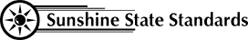MA.8.S.3.1
MA.912.A.3.11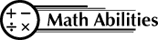Conceptual Understanding

Data Collection
Graphing Calculators
Interpret Data

Procedural Knowledge

y = mx + b
Graphing Linear Equations
Make Predictions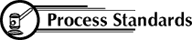Problem Solving
Reasoning
Communication
Connections
Representation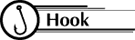Ever thought you could lose weight by the foot, not the pound?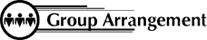Students work as a whole class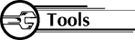• 1 bathroom scale
• 2 textbooks
• 1- 2’ X 8’ plank1. Collect the Data:
Mark the 2’ x 8’ plank into one foot increments
Place the plank on the bathroom scale and textbooks as shown below: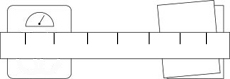Weigh a volunteer person at each of the designated locations on the plank.

 Independent Variable, X Distance from Scale (Feet) Dependent Variable, Y Weight (Pounds) 0 1 2 3 4 5 6

2. Graph the Data
Use "Distance from Scale (Feet)" as the horizontal scale and "Weight (Pounds)" as the vertical scale. Plot ordered pairs (X,Y).

Looking at your graphed points, do they appear to lie along a straight line or curve? Draw the line that best fits your data. Use the graph to answer the following:

1. Find the weight reading for the distances from the scale.
3.5 feet, _____ pounds
5.25 feet, _____ pounds
7 feet, _____ pounds

2. How much does the weight reading decrease each time the person moves another foot away from the scale?
____________________________________________________________________________________
How can you tell this from the graph?
____________________________________________________________________________________

3. What is the person’s weight when standing directly on the scale?
____________________________________________________________________________________
How can you tell this from the graph?
____________________________________________________________________________________

4. Describe in words how to determine the weight reading if you know how many feet the person is from the scale.

5. Use your description to predict the weight reading for the person when standing 4.75 feet from the scale. Show your work.

6. Describe by equation how to determine the weight reading (Y) if you know how many feet away (X) the person is from the scale:
Y= ____________________

7. Use your equation to predict the weight reading for the person when standing 6.5 feet away from the scale.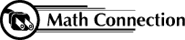As a result of this activity, students will learn how to collect, graph and interpret data.

 8th Grade Math Home > Teacher Resources > Activities > Walking the Plank: Teacher Notes